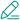# 普通写法

``````a, b, c = 1, 2, 3
if a>b:
c = a
else:
c = b
``````

# 一行表达式,为真时放if前

``````c = a if a>b else b
``````

# 二维列表，利用大小判断的0，1当作索引

``````c= [b, a][a > b]
``````

# 传说中的黑客，利用逻辑运算符进行操作，都是最简单的东西，却发挥无限能量啊

``````c = (a>b and [a] or [b])
# 改编版
c = (a>b and a or b)
``````

``````# 从前往后找，and找假，or找真
# 前真返后，
print(111 and 222)  # 222
# 前假返前
print(0 and 333)  #0

# 若x真【x】, x假,y真【y】，xy假【y】,只有前真返回前
print(111 or 222) #111
print(0 or 222) #222
print('' or 0) # 0
``````

真：a >b and a,
则a > b 为真
假：b，
则 a> b为假

`````` id_ = '12345'
# 判断长度为5或者为8
if len(id_) == 5 or len(id_) == 8:
print(id_, '------')
# 相反的表达为非5且非8
if len(id_) != 5 and len(id_) != 8:
print(id_, '+++++++')
``````

{{ comment.content }}@ {{ child.answered_user_name }}:{{ child.content }}• 微信• 如果你觉得本站很棒，可以通过扫码支付打赏哦！

•微信收款码
•支付宝收款码